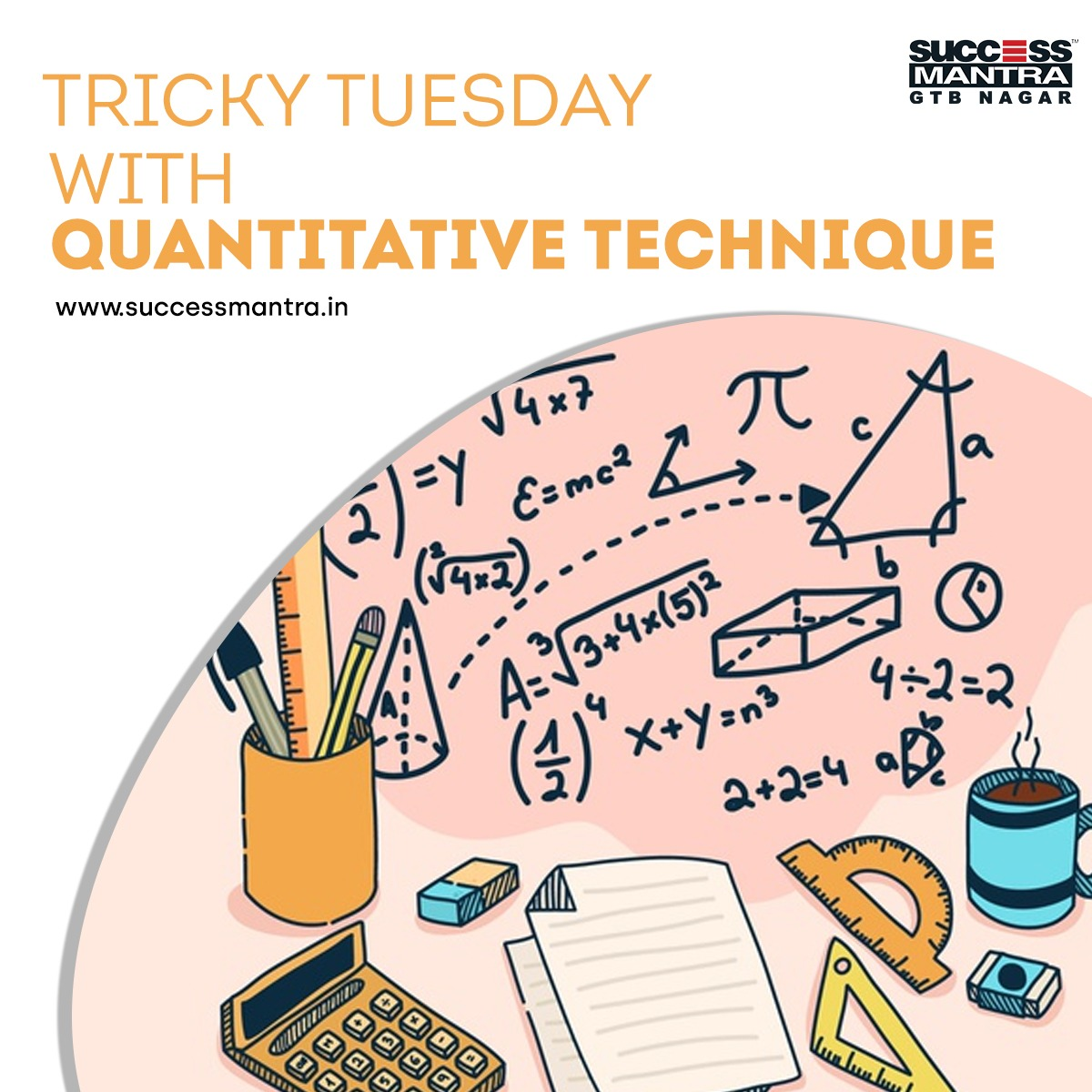## Questions On Quantitative Techniques SMQTQ0341. A boat can travel with a speed of 13 km/hr in still water. If the speed of the stream is 4 km/hr, find the time taken by the boat to go 68 km downstream.

A. 2 hours
B. 3 hours
C. 4 hours
D. 5 hours

2. A man's speed with the current is 15 km/hr and the speed of the current is 2.5 km/hr. The man's speed against the current is:

A. 8.5 km/hr
B. 9 km/hr
C. 10 km/hr
D. 12.5 km/hr

3. A boat running upstream takes 8 hours 48 minutes to cover a certain distance, while it takes 4 hours to cover the same distance running downstream. What is the ratio between the speed of the boat and speed of the water current respectively?

A. 2 : 1
B. 3 : 2
C. 8 : 3
D. Cannot be determined

4. What percentage of numbers from 1 to 70 have 1 or 9 in the unit's digit?

A. 1
B. 14
C. 20
D. 21

5. If A = x% of y and B = y% of x, then which of the following is true?

A. A is smaller than B.
B. A is greater than B
C. Relationship between A and B cannot be determined.
D. None of these